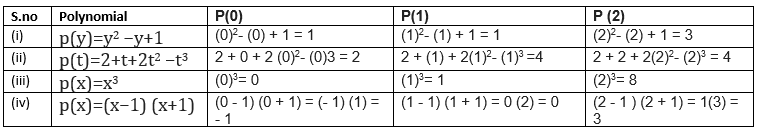# NCERT Solution for Polynomial Chapter 2 Exercise 2.1

In this page we have Class 9 Maths NCERT Solution for Polynomial Chapter 2 for Exercise 2.1 . Hope you like them and do not forget to like , social share and comment at the end of the page.

## Class 9 Maths Chapter 2 Exercise 2.1

Question 1
Find the value of the polynomial $P(x)= 5x-4x^2 +3$ at
(i) x = 0
(ii) x = -1
(iii) x = 2
Solution
(i)$P(x)= 5x-4x^2 +3$
P(0)= 0-0+3=3
(ii)$P(x)= 5x-4x^2 +3$
P(-1) =-5-4+3=-6
(iii)$P(x)= 5x-4x^2 +3$
P(2)= 10-16+3=-3

Question 2
Find p(0), p(1) and p(2) for each of the following polynomials:
(i) $p(y) = y^2 - y + 1$
(ii) $p(t) = 2 + t + 2t^2- t^3$
(iii) $p(x) = x^3$
(iv) $p(x) = (x - 1) (x + 1)$
Solution:Question 3
Verify whether the following are zeroes of the polynomial, indicated against them.
(i) $p(x) = 3x + 1$, x =- 1/3
(ii) $p(x) = 5x - \pi$, x =4/5
(iii) $p(x) = x^2 - 1$, x = 1, -1
(iv) $p(x) = (x + 1) (x - 2)$, x = - 1, 2
(v) $p(x) = x^2$ , x = 0
(vi) $p(x) = lx + m$, x = - m/l
(vii) $p(x) = 3x^2 - 1$, x =-1/√3 and 2/√3
(viii) $p(x) = 2x + 1$, x =1/2
Solution:
(i) $p(x) = 3x + 1$,x=-1/3
p(-1/3) = 3 (-1/3)+1=-1+1=0
p(-1/3) = 0 which means that-1 /3is zero of the polynomial p(x) = 3x+1.

(ii) $p(x) = 5x - \pi$, x =4/5
p(4/5) = 5(4/5)-π = 4-π
p(4/5) ≠ 0 which means that 4/5 is not zero of the polynomial $p(x) = 5x - \pi$.

(iii) $p(x) = x^2 - 1$,x = 1,- 1
p(1)=12-1=1-1=0
p(-1)=(-1)2-1=1-1=0
Both p(1) and p(-1) are equal to 0. It means that 1 and -1 are zeroes of the polynomial $p(x) = x^2 - 1$.

(iv) $p(x) = (x + 1) (x - 2)$,x=-1,2
p(-1) = (-1+1)(-1-2) = 0×-3 = 0
p(2) = (2+1)(2-2) = 3×0 = 0
Both p(-1) and p(2) are equal to 0. It means that -1 and 2 are zeroes of the polynomial $p(x) = (x + 1) (x - 2)$.

(v) $p(x) = x^2$,x = 0
p(0) = 02= 0
p(0) = 0 which means that 0 is the zero of the polynomial $p(x) = x^2$.

(vi) $p(x) = lx + m$,x =-m/
p(-m/l) =l(-m/l) + m = -m + m = 0
p(-m/l) = 0 which means that(-m/l)is zero of the polynomial $p(x) = lx + m$

(vii) $p(x) = 3x^2 - 1$, x =-1/√3 and 2/√3
p(-1/√3) = 3(-1/√3)2-1=3(1/3)-1=1-1=0
p(2/√3) = 3(2/√3)2-1 = 3 ×(4/3)-1= 4-1 = 3
p(-1/√3) = 0 which means that-1/√3is zero of the polynomial p(x) = 3x2- 1.
p(2/√3) ≠ 0 which means that2/√3is not zero of the polynomial $p(x) = 3x^2 - 1$.

(viii) p(x) = 2x + 1 ,x =1/2
p(1/2)=2 ×(1/2)+ 1=1+1=2
p(1/2) ≠ 0. It means that 1/2 is not zero of the polynomial p(x)=2x+1.

Question 4
Find the zero of the polynomial in each of the following cases:
(i) $p(x) = x + 5$
(ii) $p(x) = x - 5$
(iii) $p(x) = 2x + 5$
(iv) $p(x) = 3x - 2$
(v) $p(x) = 3x$
(vi) $p(x) = ax$, a ≠ 0
(vii) $p(x) = cx + d$, c ≠ 0, c, d are real numbers.
Solution:
Zero of a polynomial is that value of the variable at which the value of the polynomial is obtained as 0.
(i) $p(x) = x + 5$
p(x) = 0
x + 5 = 0
x = - 5
Therefore, for x = -5, the value of the polynomial is 0 and hence, x = -5 is a zero of the given polynomial.

(ii) $p(x) = x - 5$
p(x) = 0
x - 5 = 0
x = 5
Therefore, for x = 5, the value of the polynomial is0 and hence, x = 5 is a zero of the given polynomial.

(iii) $p(x) = 2x + 5$
p(x) = 0
2x + 5 = 0
2x = - 5
x = -5/2
Therefore, for x = -5/2 , the value of the polynomial is 0 and hence, x = -5/2is a zero of the given polynomial.

(iv) $p(x) = 3x - 2$
p(x) = 0
3x - 2 = 0
x=2/3, so 2/3 is the zero of the polynomial

(v) $p(x) = 3x$
p(x) = 0
3x = 0
x = 0
So x=0 is the zero of the polynomial

(vi) $p(x) = ax$
p(x) = 0
ax = 0
x = 0
Therefore, for x = 0, the value of the polynomial is 0 and hence, x = 0 is a zero of the given polynomial.

(vii) $p(x) = cx + d$
p(x) = 0
cx+ d = 0
x=-d/c

## Practice Questions

Write whether the following statements are True or False. Justify your answer.
(i) A binomial can have atmost two terms
(ii) Every polynomial is a binomial
(iii) A binomial may have degree 5
(iv) Zero of a polynomial is always 0
(v) A polynomial cannot have more than one zero
(vi) The degree of the sum of two polynomials each of degree 2 is always 4

## Summary

1. NCERT solutions for class 9 maths chapter 2 Exercise 2.1 has been prepared by Expert with utmost care. If you find any mistake.Please do provide feedback on mail. You can download the solutions as PDF in the below Link also
2. This chapter 2 has total 6 Exercise 2.1 ,2.2,2.3 ,2.4 and 2.5. This is the First exercise in the chapter.You can explore previous exercise of this chapter by clicking the link below